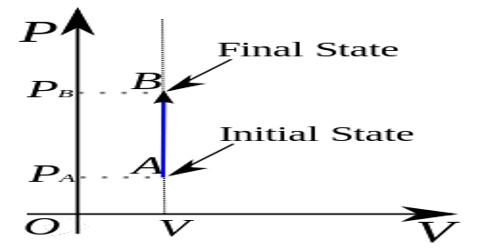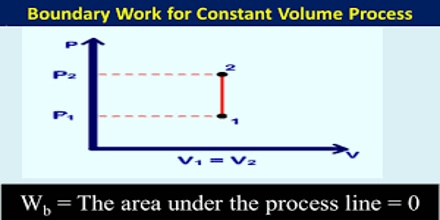Chemistry

# Constant Volume Processes in ThermodynamicsConstant Volume Processes

It the thermodynamic process is carried out at constant volume, w = 0, since dV = zero. Hence the first law equation takes the form,

q = dU … … … (1)

The heat absorbed or evolved directly changes the internal energy. For example, if a chemical reaction is carried out in a stainless-steel pressurized container the heat produced must increase the internal energy by raising the temperature since the volume is fixed.Effects of Pressure Changes on Fluid Properties: The predominant effect of an increase in pressure in a compressible fluid, such as a gas, is an increase in the density of the fluid. An increase in the pressure of an incompressible fluid will not have a significant effect on the density. For example, increasing the pressure of 100°Fwater from 15 psia to 15,000 psia will  only increase the density by approximately 6%.Therefore, in engineering calculations, it is assumed that incompressible fluids’ density remain constant.